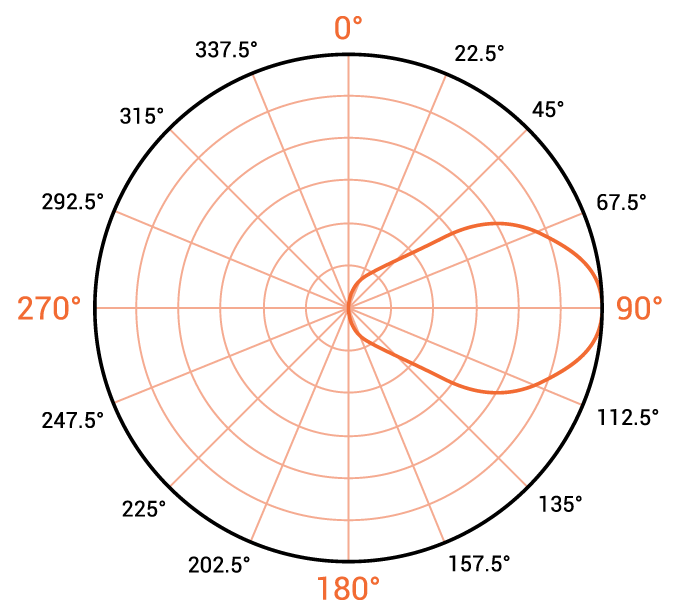# EIRP Calculator

## A helpful tool for computing the Effective Isotropic Radiated Power (EIRP) of an antenna

(dB)
(dBi)

(dB) decibel

### Overview

An antenna's effective isotropic radiated power (EIRP) is its power measured in one direction. It is named as such because it is the power an isotropic (perfectly omnidirectional) antenna would have to radiate to achieve the same value. This calculator is designed to compute the EIRP given the transmitter's radiated power, the loss introduced by the cable from the transmitter to the antenna, and the antenna's gain. To use this tool, enter the transmitter's output power and choose the appropriate unit of measurement (dBm, dBw, milliwatts or watts). Also provide the cable loss and the antenna's gain and press the calculate button.### Equation

$$EIRP = P_{T} - L_{C} + G_{TX}$$

Where:

$$EIRP$$ = effective isotropic radiated power in dB

$$P_{T}$$ = radiated power of the antenna in dbW

$$L_{C}$$ = signal loss caused by the cable from the transmitter circuit to the antenna in dB

$$G_{TX}$$ = gain of the transmitting antenna in dB

### Applications

The EIRP (also, equivalent isotropic radiated power) is the equivalent power that an isotropic antenna needs to radiate to achieve the same power density in a single direction at a given point as another antenna. For instance, if a given transmit antenna has a power gain of 20, the power density from the antenna is 20 times greater than it would have been had the antenna been an isotropic one. An isotropic antenna would have to radiate 20 times as much power to achieve the same power density. Therefore, the given antenna effectively radiates 20 times as much power as an isotropic antenna with the same input power and efficiency.